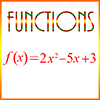# Basics of FunctionsA function is a mathematical rule that associates every element in one set – called the domain of the function – with a unique element in some other (or possibly the same) set, called the range. For example, if the function named f is defined by the polynomial 3x + 1, then both the domain and the range of the function f is the set of real numbers. Thus, if x is any real number, then the function f associates x with the value 3x + 1. This is written as f(x) = 3x + 1. This is said “f of x = 3x + 1.” Different values for x and f(x) are shown in the following table:

 x f(x) = 3x + 1 0 1 2 7 3 10 -1 -2 -2 -5

Thus, if x is 0, then f(x) is 1; if x is 2, f(x) is 7, etc. We can shorten this by saying, f(0) = 1, f(2) = 7, etc. Notice that we replace the x in the expression f(x) and in the polynomial 3x + 1 with the number 0 or the number 2, etc., so, for example, f(2) = 3·2 + 1 = 6 + 1 = 7.

## Examples of Functions

Absolute value is a function which for any real number x gives the distance x is from 0. If x is a positive real number, then its absolute value, |x|, is x. For example, if x is 3, then |x| = |3| = 3. If x is a negative real number, then |x| = -x. For example, if x is -3, then |x| = |-3| = -(-3) = 3. Notice that both 3 and -3 are the same distance, 3, from 0.

A sequence is a function whose domain is usually the set of non-negative integers. Its range can vary, but is usually some subset of the real numbers. It is often shown as a series of numbers with the property that each number after the first has a particular relationship to its immediate predecessor.

Example: In this sequence, 4, 7, 10, 13, … each number is 3 greater than the number preceding it. This is an example of an arithmetic sequence. The value of each number in a sequence can be determined if we know the value of the first number and the rule that determines for any given number what the next number will be.

Statistical functions return a number which is calculated from a set of values. The particular function is intended to identify something about the set as a whole. Commonly used statistical functions include the mean, median, mode, range of a set of values, variance, and standard deviation.

Sample Function Problems